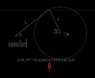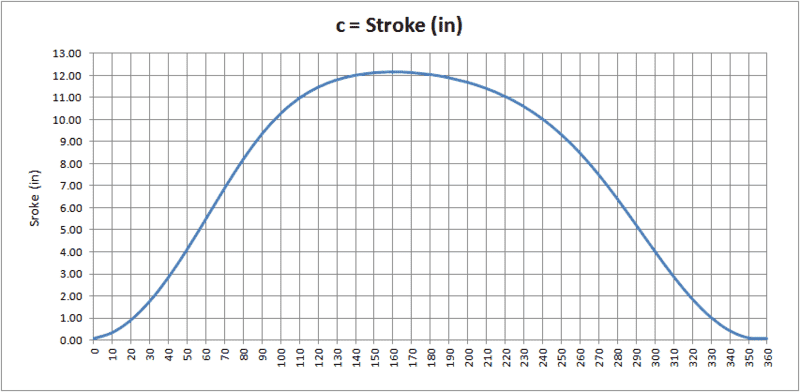# Offset slider-crank mechanism

• aleset

#### aleset

I have a offset slider crank mechanism. Of which I know all the geometrical data, because I measure them .

I do not understand how I can calculate the rotation angle of the connecting rod knowing the stroke.

in my formula I have the angle b. I do not understand how to eliminate it from the formula

regardes

#### Attachments

•ang.JPG
18.1 KB · Views: 666

I have a offset slider crank mechanism. Of which I know all the geometrical data, because I measure them .

I do not understand how I can calculate the rotation angle of the connecting rod knowing the stroke.

in my formula I have the angle b. I do not understand how to eliminate it from the formula

regardes
You have two unknowns, ##a## and ##b##, but only one equation. If you want to solve for ##b##, you need one more equation involving ##a## and ##b##.

thanks thinch.

is there an alternative formula that relates the angle "a" with the stroke "c" without knowing the angle "b"?

Wrong. See my posts 6 and 7, below.

1. Center of rotation of crank. Call this 'γ'
3. Angle of crank, 'α'
4. Length of connecting rod, 'L'
5. Location and orientation of stroke 'C' centerline
I'm doing this in rectangular coordinates because I find it easier to think about.

• With the first three knowns ('γ', 'r', 'α') you find crank location, the intersection of 'r' and 'L'. Call this point 'δ'
• Find the point(s) on a circle centered at 'δ', of radius 'L', that intersect the line that describes the stroke 'C'
• You now have the location of three triangle vertices, the length of two sides, and the third side is easily calculable. It should be (relatively) easy to find the one missing angle.
• Angle 'b' is arctan({ΔY of "L"} / {ΔX of "L"})
EDIT: strikeout and added Angle 'b' calc

https://math.stackexchange.com/ques...intersections-of-a-straight-line-and-a-circle
https://www.mathportal.org/calculators/analytic-geometry/circle-line-intersection-calculator.php

https://math.stackexchange.com/ques...ind-the-points-at-which-two-circles-intersect
http://www.ambrsoft.com/TrigoCalc/Circles2/circle2intersection/CircleCircleIntersection.htm

Cheers,
Tom

Last edited:
I'm sorry. I misread the original problem.I solved for 'b' when 'a' is known, where you seem to want 'a' when 'b' is known.
I will have to think on that for a while.

Just to verify so I don't mess up again, are these known?
2. Connecting rod length 'L'
3. Offset of stroke path from crank center 'e'
4. Angle 'b'
5. Horizontal Position of left end of Connecting rod relative to crank center when 'b' is known

Tom

Last edited:
Think I've got it this time.

Find angle 'a'.

Knowns:
1. Rotational center of crank shaft
3. Connecting rod length 'L'
4. Path of stroke (centerline)
5. Angle 'b'

Solution:

If you draw your figure with α = 90° you will see that another substitution for β is: asin ((r sin α) + e)/L) for solving for c based upon an input α value; but, cannot be reconfigured to solve for α based upon a c input value.

Edit: I have now realized something I should have seen earlier. You cannot have an equation to find an angle α from the stroke location c because there are two possible α angles in the crank rotation for anyone stroke distance c.=; as clearly illustrated in the below graph using sample L, r and e values for this problem.Last edited: